# Insertion Sort in Java Programming

###### Sorting in Java

In this lesson, we will understand what is Insertion Sort in Java Programming along with some examples.

## What is Insertion Sort in Java?

An Insertion Sort is a sorting technique used in java to sort elements of an array in ascending or descending order.

In this sorting technique, we assume that the first number in the array is in the sorted section and the rest of all the other numbers in the array are in the unordered section. Now we will pick each number from the unsorted section and insert that number at a proper position in the sorted section by shifting the numbers towards the right.

To clearly understand the insertion sorting technique, let's go through its algorithm step by step with an example.## Insertion Sort Visualization

Suppose we have seven numbers stored in an array as shown below.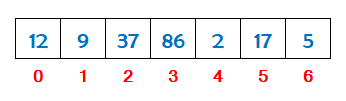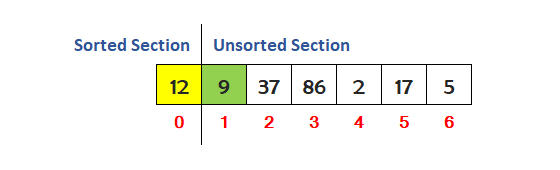First, select the number 9 from the unsorted section of the array and find its proper position in the sorted section of the array. The proper position of 9 is at index 0. So, we have to shift the numbers from the sorted section of the array towards the right to insert 9 at it proper position.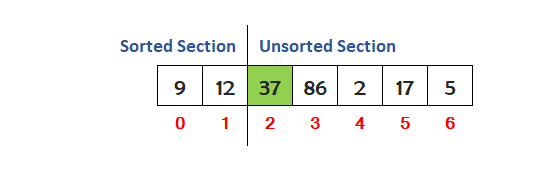Now, select the number 37 from the unsorted section of the array and find its proper position in the sorted section of the array. We can see that the number is already at it proper position. So leave it.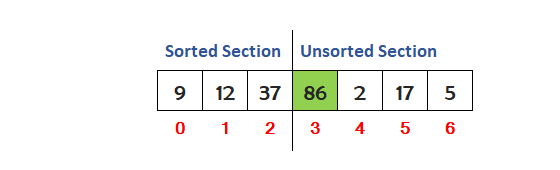Now, select the number 86 from the unsorted section of the array and find its proper position in the sorted section of the array. We can see that the number is already at it proper position. So leave it.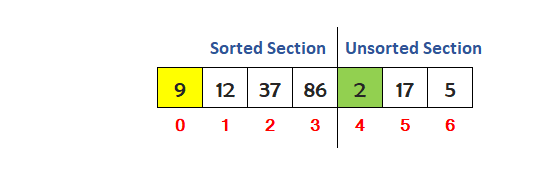Now, select the number 2 from the unsorted section of the array and find its proper position in the sorted section of the array. The proper position of 2 is at index 0. So, we have to shift the numbers from the sorted section of the array towards the right to insert 2 at it proper position.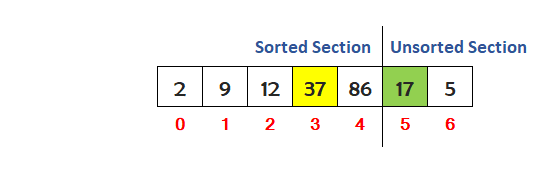Now, select the number 17 from the unsorted section of the array and find its proper position in the sorted section of the array. The proper position of 17 is at index 3. So, we have to shift the numbers from the sorted section of the array towards the right to insert 17 at it proper position.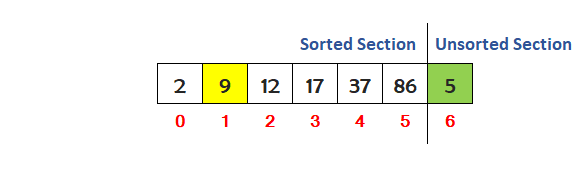Now, select the number 5 from the unsorted section of the array and find its proper position in the sorted section of the array. The proper position of 5 is at index 1. So, we have to shift the numbers from the sorted section of the array towards the right to insert 5 at it proper position.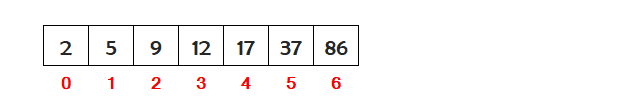So we can see that the entire array has sorted in ascending order.

## Algorithm for Insertion Sort

An algorithm is the steps used to implement the insertion sort in a java program.

Assume we have N numbers stored in an array. To implement the insertion sort on N numbers, the steps are as follows.

• Define an array to store N numbers for insertion sort. Suppose we have defined an array with the name num.
• Run an outer loop i from 1 to N to repeat the process of insertion sort.
• Store the number num[i] to be inserted at proper place in variable x.
• Run an inner while loop j inside the body of the outer loop i for insertion sort from i-1 to 0.
• Now check if the value of x is less than value of num[j] then shift the number num[j] towards right else break the inner loop j.
• Outside the body of inner loop j insert the value of x at num[j+1] position.
• Now print the sorted array on the screen outside the body of the outer loop i.

## Java Program for Insertion Sort

A Java program to sort an array of numbers in ascending order using the insertion sort technique.

#### Insertion Sort (List of Numbers in Ascending Order)

``````public class InsertionSortAscending
{
public static void main(String args[])
{
// define an array
int num[]= {12,9,37,86,2,17,5};
int i,j,x;

System.out.println("Array before Insertion Sort");
for(i=0; i<num.length; i++)
{
System.out.print(num[i]+" ");
}

// run an outer loop i from 1 to num.length to repeat the process of insertion sort
for(i=0; i<num.length; i++)
{
// x to be inserted at proper place
x=num[i];

// run an inner while loop j for insertion sort from i-1 to 0
j=i-1;
while(j>=0)
{
// now check if the value of x is less than num[j] then shift the number num[j] towards right else break the inner loop j
if(x<num[j])
{
num[j+1]=num[j];
}
else
{
break;
}
j=j-1;
}

// outside the body of inner loop j insert the value of x at num[j+1] position
num[j+1]=x;
}

// print the sorted array
System.out.print("\n\nArray after Insertion Sort\n");
for(i=0; i<num.length; i++)
{
System.out.print(num[i]+" ");
}
}
}``````

#### Output

```Array before Insertion Sort
12 9 37 86 2 17 5

Array after Insertion Sort
2 5 9 12 17 37 86```

A Java program to sort an array of numbers in descending order using the insertion sort technique.

#### Insertion Sort (List of Numbers in Descending Order)

``````public class InsertionSortDescending
{
public static void main(String args[])
{
// define an array
int num[]= {12,9,37,86,2,17,5};
int i,j,x;

System.out.println("Array before Insertion Sort");
for(i=0; i<num.length; i++)
{
System.out.print(num[i]+" ");
}

// run an outer loop i from 1 to num.length to repeat the process of insertion sort
for(i=0; i<num.length; i++)
{
// x to be inserted at proper place
x=num[i];

// run an inner while loop j for insertion sort from i-1 to 0
j=i-1;
while(j>=0)
{
// now check if the value of x is greater than num[j] then shift the number num[j] towards right else break the inner loop j
if(x>num[j])
{
num[j+1]=num[j];
}
else
{
break;
}
j=j-1;
}

// outside the body of inner loop j insert the value of x at num[j+1] position
num[j+1]=x;
}

// print the sorted array
System.out.print("\n\nArray after Insertion Sort\n");
for(i=0; i<num.length; i++)
{
System.out.print(num[i]+" ");
}
}
}``````

#### Output

```Array before Insertion Sort
12 9 37 86 2 17 5

Array after Insertion Sort
86 37 17 12 9 5 2```

A Java program to sort an array of strings in ascending order using the insertion sort technique.

#### Insertion Sort (List of Strings in Ascending Order)

``````public class InsertionSortStringAscending
{
public static void main(String args[])
{
// define an array
String num[]= {"Squirrel","Dog","Panda","Lion","Bear","Tiger","Rabbit"};
String x;
int i,j;

System.out.println("Array before Insertion Sort");
for(i=0; i<num.length; i++)
{
System.out.print(num[i]+" ");
}

// run an outer loop i from 1 to num.length to repeat the process of insertion sort
for(i=0; i<num.length; i++)
{
// x to be inserted at proper place
x=num[i];

// run an inner while loop j for insertion sort from i-1 to 0
j=i-1;
while(j>=0)
{
// now check if the value of x is less than num[j] then shift the string num[j] towards right else break the inner loop j
if(x.compareToIgnoreCase(num[j])<0)
{
num[j+1]=num[j];
}
else
{
break;
}
j=j-1;
}

// outside the body of inner loop j insert the value of x at num[j+1] position
num[j+1]=x;
}

// print the sorted array
System.out.print("\n\nArray after Insertion Sort\n");
for(i=0; i<num.length; i++)
{
System.out.print(num[i]+" ");
}
}
}``````

#### Output

```Array before Insertion Sort
Squirrel  Dog  Panda  Lion  Bear  Tiger  Rabbit

Array after Insertion Sort
Bear  Dog  Lion  Panda  Rabbit  Squirrel  Tiger```

## Insertion Sort Program Explanation

The explanation of the above program (List of Numbers in Ascending Order) is given below in details.

#### Explanation

```Array before Insertion Sort
12 9 37 86 2 17 5

The outer loop i starts running from 1 to 6

When i = 1
x = 9

The inner while loop j starts running from 0 to 0

When j = 0   Check if 9<12      Yes 9<12 then shift the number 12 towards right
Array is : 12 12 37 86 2 17 5

The inner loop ends. Now insert the value of x=9 at position 0
Array is : 9 12 37 86 2 17 5

When i = 2
x = 37

The inner while loop j starts running from 1 to 0

When j = 1   Check if 37<12     No then break the inner loop j

The inner loop ends. Now insert the value of x=37 at position 2
Array is : 9 12 37 86 2 17 5

When i = 3
x = 86

The inner while loop j starts running from 2 to 0

When j = 2   Check if 86<37     No then break the inner loop j

The inner loop ends. Now insert the value of x=86 at position 3
Array is : 9 12 37 86 2 17 5

When i = 4
x = 2

The inner while loop j starts running from 3 to 0

When j = 3   Check if 2<86      Yes 2<86 then shift the number 86 towards right
Array is : 9 12 37 86 86 17 5

When j = 2   Check if 2<37      Yes 2<37 then shift the number 37 towards right
Array is : 9 12 37 37 86 17 5

When j = 1   Check if 2<12      Yes 2<12 then shift the number 12 towards right
Array is : 9 12 12 37 86 17 5

When j = 0   Check if 2<9      Yes 2<9 then shift the number 9 towards right
Array is : 9 9 12 37 86 17 5

The inner loop ends. Now insert the value of x=2 at position 0
Array is : 2 9 12 37 86 17 5

When i = 5
x = 17

The inner while loop j starts running from 4 to 0

When j = 4   Check if 17<86      Yes 17<86 then shift the number 86 towards right
Array is : 2 9 12 37 86 86 5

When j = 3   Check if 17<37      Yes 17<37 then shift the number 37 towards right
Array is : 2 9 12 37 37 86 5

When j = 2   Check if 17<12     No then break the inner loop j

The inner loop ends. Now insert the value of x=17 at position 3
Array is : 2 9 12 17 37 86 5

When i = 6
x = 5

The inner while loop j starts running from 5 to 0

When j = 5   Check if 5<86      Yes 5<86 then shift the number 86 towards right
Array is : 2 9 12 17 37 86 86

When j = 4   Check if 5<37      Yes 5<37 then shift the number 37 towards right
Array is : 2 9 12 17 37 37 86

When j = 3   Check if 5<17      Yes 5<17 then shift the number 17 towards right
Array is : 2 9 12 17 17 37 86

When j = 2   Check if 5<12      Yes 5<12 then shift the number 12 towards right
Array is : 2 9 12 12 17 37 86

When j = 1   Check if 5<9      Yes 5<9 then shift the number 9 towards right
Array is : 2 9 9 12 17 37 86

When j = 0   Check if 5<2     No then break the inner loop j

The inner loop ends. Now insert the value of x=5 at position 1
Array is : 2 5 9 12 17 37 86

The outer loop ends

Array after Insertion Sort
2 5 9 12 17 37 86```